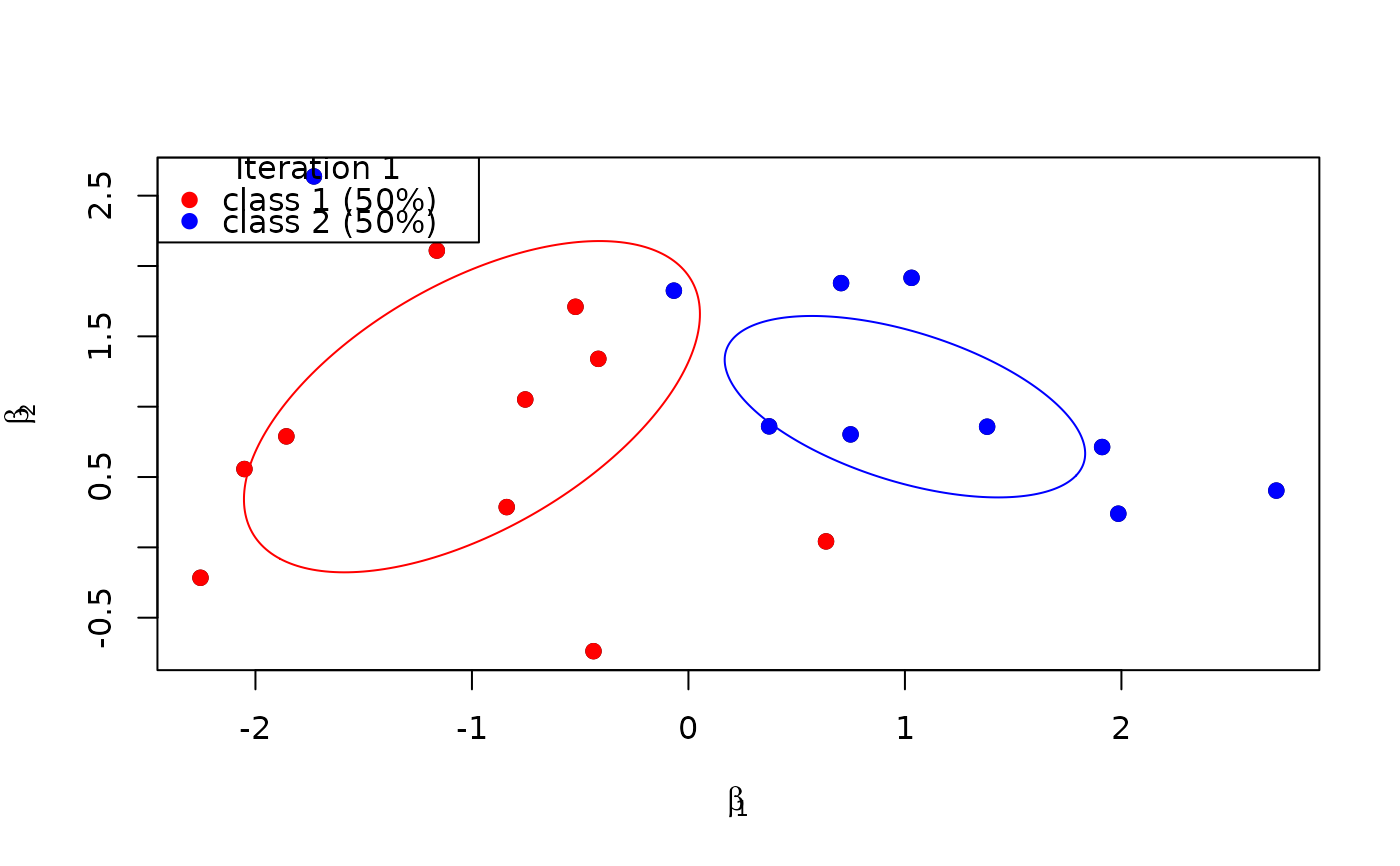This function plots the allocation of decision-maker specific coefficient vectors beta given the allocation vector z, the class means b, and the class covariance matrices Omega.

## Usage

plot_class_allocation(beta, z, b, Omega, ...)

## Arguments

beta

The matrix of the decision-maker specific coefficient vectors of dimension P_r x N. Set to NA if P_r = 0.

z

The vector of the allocation variables of length N. Set to NA if P_r = 0.

b

The matrix of class means as columns of dimension P_r x C. Set to NA if P_r = 0.

Omega

The matrix of class covariance matrices as columns of dimension P_r*P_r x C. Set to NA if P_r = 0.

...

Optional visualization parameters:

• colors, a character vector of color specifications,

• perc, a numeric between 0 and 1 to draw the perc percentile ellipsoids for the underlying Gaussian distributions (perc = 0.95 per default),

• r, the current iteration number of the Gibbs sampler to be displayed in the legend,

• sleep, the number of seconds to pause after plotting.

## Value

No return value. Draws a plot to the current device.

## Details

Only applicable in the two-dimensional case, i.e. only if P_r = 2.

## Examples

b <- matrix(c(-1,1,1,1), ncol = 2)
Omega <- matrix(c(0.8,0.5,0.5,1,0.5,-0.2,-0.2,0.3), ncol = 2)
z <- rep(1:2, each = 10)
beta <- sapply(z, function(z) rmvnorm(mu = b[,z], Sigma = matrix(Omega[,z], ncol = 2)))
RprobitB:::plot_class_allocation(beta = beta, z = z, b = b, Omega = Omega,
colors = c("red","blue"), perc = 0.5, r = 1)